Courses

# Syllogism MCQ 2

## 20 Questions MCQ Test Logical Reasoning for CLAT | Syllogism MCQ 2

Description
This mock test of Syllogism MCQ 2 for CLAT helps you for every CLAT entrance exam. This contains 20 Multiple Choice Questions for CLAT Syllogism MCQ 2 (mcq) to study with solutions a complete question bank. The solved questions answers in this Syllogism MCQ 2 quiz give you a good mix of easy questions and tough questions. CLAT students definitely take this Syllogism MCQ 2 exercise for a better result in the exam. You can find other Syllogism MCQ 2 extra questions, long questions & short questions for CLAT on EduRev as well by searching above.
QUESTION: 1

### In this question six statements are followed by four answer choices. Each of the answer choices has a combination of three statements  from the given statements. You are required to identify the answer choice in which the given statements are logically related and the third statement is a conclusion derived from the first two statements. Q.  A. Some boats are drawers. B. Some boats are brown. C. All drawers are brown. D. All boats are brown. E. All brown are boats. F. Some brown are not boats.

Solution:

The correct option is B.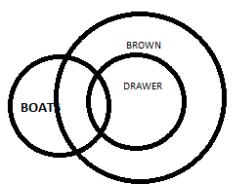QUESTION: 2

### In this question six statements are followed by four answer choices. Each of the answer choices has a combination of three statements  from the given statements. You are required to identify the answer choice in which the given statements are logically related and the third statement is a conclusion derived from the first two statements. Q.   A. All black are Rats. B. Some dogs are Rats. C. Some Rats are black. D. All Rats are dogs. E. All dogs are Rats. F. Some dogs are black.

Solution:

The correct option is D.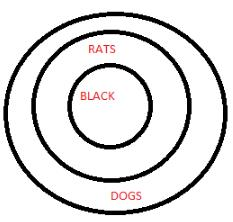QUESTION: 3

### In this question six statements are followed by four answer choices. Each of the answer choices has a combination of three statements  from the given statements. You are required to identify the answer choice in which the given statements are logically related and the third statement is a conclusion derived from the first two statements. Q.   A. Some square are round. B. All square are rectangle. C. Some round are rectangle. D. All round are square. E. All rectangle are square. F. Some rectangle are square.

Solution:
QUESTION: 4

In this question six statements are followed by four answer choices. Each of the answer choices has a combination of three statements  from the given statements. You are required to identify the answer choice in which the given statements are logically related and the third statement is a conclusion derived from the first two statements.

Q.
A. Some social Area.

B. No Area is radio.

C. Some Area may not be social.

D. All Area are radio.

E. All social are radio.

F. Some social are not Area.

Solution:
QUESTION: 5

In this question six statements are followed by four answer choices. Each of the answer choices has a combination of three statements  from the given statements. You are required to identify the answer choice in which the given statements are logically related and the third statement is a conclusion derived from the first two statements.

Q.
A. All Region is hyper.

B. All hyper is sad.

C. All Region is sad.

D. Some hyper is Region.

E. Some Region is sad.

F. Some hyper is sad.

Solution:
QUESTION: 6

In this question six statements are followed by four answer choices. Each of the answer choices has a combination of three statements  from the given statements. You are required to identify the answer choice in which the given statements are logically related and the third statement is a conclusion derived from the first two statements.

Q.
A. All Mammals are people.

B. Some Mammals are people.

C. Some people are happy.

D. Some people are unhappy.

E. All people are happy.

F. All happy are Mammals.

Solution:
QUESTION: 7

In this question six statements are followed by four answer choices. Each of the answer choices has a combination of three statements  from the given statements. You are required to identify the answer choice in which the given statements are logically related and the third statement is a conclusion derived from the first two statements.

Q.
A. Some gases is solid.

B. All gases is water.

C. Some water is gases.

D. All water is solid.

E. All solid is gases.

F. Some water is solid.

Solution: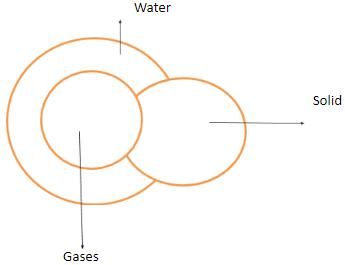QUESTION: 8

In this question six statements are followed by four answer choices. Each of the answer choices has a combination of three statements  from the given statements. You are required to identify the answer choice in which the given statements are logically related and the third statement is a conclusion derived from the first two statements.

Q.
A. Some green is black.

B. Some black is green.

C, Some sky is black.

D. All green is black.

E. All black is sky.

F. All sky is green.

Solution:
QUESTION: 9

In this question six statements are followed by four answer choices. Each of the answer choices has a combination of three statements  from the given statements. You are required to identify the answer choice in which the given statements are logically related and the third statement is a conclusion derived from the first two statements.

Q.
A. All dancer are silver.

B. Some Helen are silver.

C. Some dancer may not be Helen.

D. Some dancer are Helen.

E. All silver are Helen.

F. All dancer are Helen.

Solution:

The correct option is B.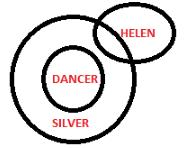QUESTION: 10

In this question six statements are followed by four answer choices. Each of the answer choices has a combination of three statements  from the given statements. You are required to identify the answer choice in which the given statements are logically related and the third statement is a conclusion derived from the first two statements.

Q.
A. All money is lucky.

B. Some money is good.

C. All money is good.

D. Some lucky is money.

E. All lucky is good.

F. Some lucky is good.

Solution:

The correct option is D.

For clarification refer to the image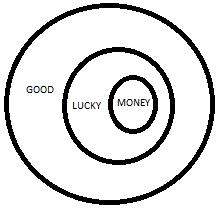QUESTION: 11

In this question six statements are followed by four answer choices. Each of the answer choices has a combination of three statements  from the given statements. You are required to identify the answer choice in which the given statements are logically related and the third statement is a conclusion derived from the first two statements.

Q.
A. Some  blue are tree.

B. Some paper are not blue.

C. All tree are paper.

D. Some blue are paper.

E. All paper are blue.

F. All blue are paper.

Solution:

B is the correct option. The ACD  all together makes  a sensible statement set. A. Some  blue are tree. C. All tree are paper. D. Some blue are paper.

QUESTION: 12

In this question six statements are followed by four answer choices. Each of the answer choices has a combination of three statements  from the given statements. You are required to identify the answer choice in which the given statements are logically related and the third statement is a conclusion derived from the first two statements.

Q.
A. Some toes are taps.

B. Some taps are hands.

C. All toes are taps.

D. All toes are hands.

E. Some taps are toes.

F. Some hands are taps.

Solution:

The correct option is A.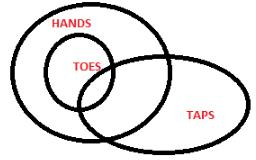QUESTION: 13

In this question six statements are followed by four answer choices. Each of the answer choices has a combination of three statements  from the given statements. You are required to identify the answer choice in which the given statements are logically related and the third statement is a conclusion derived from the first two statements.

Q.
A. All pen are pencil.

B. All bin are pencil.

C. Some pencil are pen.

D. All pencil are bin.

E. Some bin are pencil.

F. Some bin are pen.

Solution:

A is the correct option. The ADF all together makes  a sensible statement set. A. All pen are pencil. D. All pencil are bin. F. Some bin are pen.

QUESTION: 14

In this question six statements are followed by four answer choices. Each of the answer choices has a combination of three statements  from the given statements. You are required to identify the answer choice in which the given statements are logically related and the third statement is a conclusion derived from the first two statements.

Q.
A. Some happy are good.

B. Some happy are bad.

C. No good is bad.

D. No happy is good.

E. All bad are good.

F. Some happy are not bad.

Solution:

B is the correct option. The ACF  all together makes  a sensible statement set. A. Some happy are good. C. No good is bad. F. Some happy are not bad.

QUESTION: 15

In this question six statements are followed by four answer choices. Each of the answer choices has a combination of three statements  from the given statements. You are required to identify the answer choice in which the given statements are logically related and the third statement is a conclusion derived from the first two statements.

Q.
A. Some treats are tasty.

B. Some treats may not be sweet.

C. All sweet are treats

D. All sweet are tasty.

E. All treats are tasty.

F. Some treats are sweet.

Solution:

C is the correct option.The ADB all together makes  a sensible statement set. A. Some treats are tasty. D. All sweet are tasty. B. Some treats may not be sweet.

QUESTION: 16

In this question six statements are followed by four answer choices. Each of the answer choices has a combination of three statements  from the given statements. You are required to identify the answer choice in which the given statements are logically related and the third statement is a conclusion derived from the first two statements.

Q.
A. Some bosses are analytical.

B. All analytical bosses are intelligent.

C. Mr. Rohit is a boss.

D. Mr. Rohit is analytical.

E. Mr. Rohit is intelligent.

F. All bosses are intelligent.

Solution:

A is the correct option.The CFE all together makes  a sensible statement set. C. Mr. Rohit is a boss.F. All bosses are intelligent.E. Mr. Rohit is intelligent.

QUESTION: 17

In this question six statements are followed by four answer choices. Each of the answer choices has a combination of three statements  from the given statements. You are required to identify the answer choice in which the given statements are logically related and the third statement is a conclusion derived from the first two statements.

Q.
A. All grooms are brooms.

B. Only brooms are toffees.

C. All brooms are cakes.

D. All grooms are toffees.

E. All brooms are toffees.

F. All toffees are cakes.

Solution:
QUESTION: 18

In this question six statements are followed by four answer choices. Each of the answer choices has a combination of three statements  from the given statements. You are required to identify the answer choice in which the given statements are logically related and the third statement is a conclusion derived from the first two statements.

Q.
A.  All mechanics are controllable.

B. All mechanics are definable.

C. All devices need contraptions.

D. All mechanics need instruments.

E. All devices need instruments.

F. All mechanics are devices.

Solution:

Option C is the correct Answer.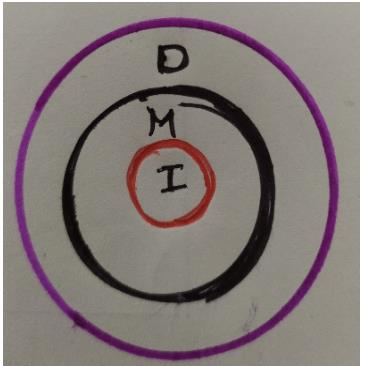QUESTION: 19

In this question six statements are followed by four answer choices. Each of the answer choices has a combination of three statements  from the given statements. You are required to identify the answer choice in which the given statements are logically related and the third statement is a conclusion derived from the first two statements.

Q.
A. Some persons are bold.

B. All bold persons are progressive.

C. Titanic is a persons.

D. Titanic is bold.

E. Titanic is progressive.

F. All persons are progressive.

Solution:
QUESTION: 20

In this question six statements are followed by four answer choices. Each of the answer choices has a combination of three statements  from the given statements. You are required to identify the answer choice in which the given statements are logically related and the third statement is a conclusion derived from the first two statements.

Q.
A. All monkeys are civilised.

B. Only the decent are monkeys.

C. All those who are decent are civilized.

D. All those worth worshipping are monkeys.

E. All those who are decent are monkeys.

F. All those worth worshipping are decent.

Solution: